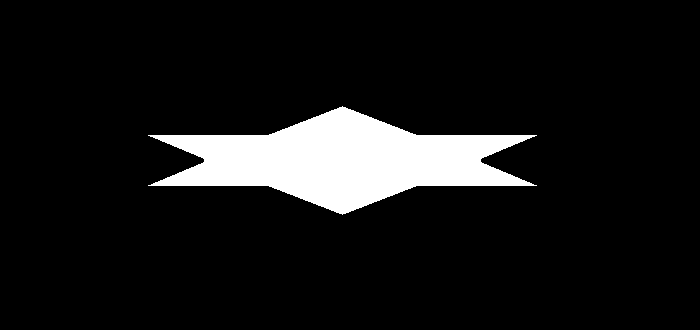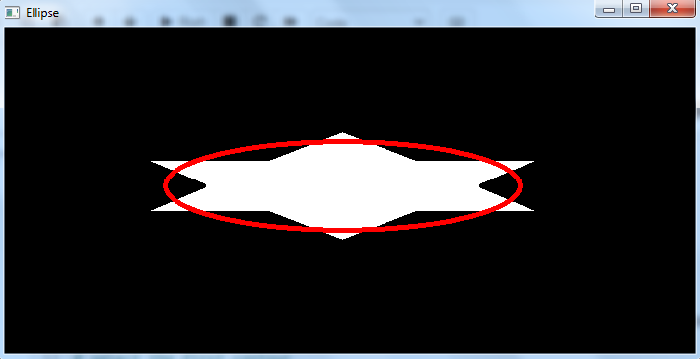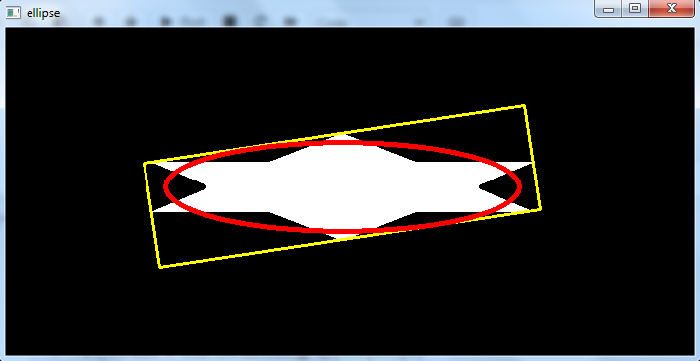# How to fit the ellipse to an object in an image using OpenCV Python?

We can fit an ellipse to an object using the function cv2.fitEllipse(). The ellipse is inscribed in a rotated rectangle. The rotated rectangle is a bounding rectangle with minimum area enclosing the object.

## Syntax

The syntax used for this function is −

ellipse = cv2.fitEllipse(cnt)


Where, "cnt" is the contour points. It is represented as an array of contour points.

Output − It returns a tuple of tuples in ((x,y), (majorAxis, minorAxis), angle) format. (x,y) is the coordinates of center and (majorAxis, minorAxis) is the lengths of minor and major axes and angle is the rotation angle of the ellipse.

To draw an ellipse on the input image, we use the following function −

cv2.ellipse(img,ellipse, (0,0,255), 3)


## Steps

You can use the following steps to fit an ellipse to an object −

Import the required library. In all the following Python examples, the required Python library is OpenCV. Make sure you have already installed it.

import cv2


img = cv2.imread('star1.png')
gray = cv2.cvtColor(img, cv2.COLOR_BGR2GRAY)


Apply thresholding on the grayscale image to create a binary image. Adjust the second argument for better contour detection.

ret,thresh = cv2.threshold(gray,150,255,0)


Find the contours in the image using cv2.findContours() function.

contours, _ = cv2.findContours(thresh, cv2.RETR_TREE, cv2.CHAIN_APPROX_SIMPLE)


Select a contour "cnt" or loop over all contours. Fit the ellipse to the object contour "cnt" using "cv2.fitEllipse(cnt)" function.

cnt = contours
ellipse = cv2.fitEllipse(cnt)


Draw the ellipse on the input image.

cv2.ellipse(img,ellipse, (0,0,255), 3)


Display the image with the drawn Convex Hull.

cv2.imshow("Ellipse", img)
cv2.waitKey(0)
cv2.destroyAllWindows()


Let's have a look at some examples for more clear understanding.

We use the following image as the input file for the examples below.## Example 1

In the Python program below, we detect the contours of the object in the image and find the ellipse fitting the object. We draw the ellipse on the input image.

# import required libraries
import cv2
import numpy as np

gray = cv2.cvtColor(img, cv2.COLOR_BGR2GRAY)

# apply thresholding to convert grayscale to binary image
ret,thresh = cv2.threshold(gray,150,255,0)

# find the contours
contours,hierarchy = cv2.findContours(thresh, cv2.RETR_TREE,cv2.CHAIN_APPROX_SIMPLE)
print("Number of contours detected:", len(contours))

# select the first contour
cnt = contours

# fit the ellipse to the selected object
ellipse = cv2.fitEllipse(cnt)

# draw the ellipse on the input image
cv2.ellipse(img,ellipse, (0,0,255), 3)

# display the image with an ellipse drawn on it.
cv2.imshow("Ellipse", img)
cv2.waitKey(0)
cv2.destroyAllWindows()


## Output

When you execute the above code, it will produce the following output −When you execute the above code, it will produce the following output −

Number of contours detected: 1


And we get the following output window −The ellipse fitting the detected object is drawn in red color.

Let's find the rotated rectangle in which the ellipse is inscribed.

## Example 2

In this Python program, we detect the contours of an object in the image and find the ellipse fitting the object. We also find the rotated rectangle in which the ellipse is inscribed. We draw the ellipse and the rotated rectangle on the input image.

# import required libraries
import cv2
import numpy as np

gray = cv2.cvtColor(img, cv2.COLOR_BGR2GRAY)

# apply thresholding to convert grayscale to binary image
ret,thresh = cv2.threshold(gray,150,255,0)

# find the contours
contours,hierarchy = cv2.findContours(thresh, cv2.RETR_TREE,cv2.CHAIN_APPROX_SIMPLE)
print("Number of contours detected:", len(contours))

# select the first contour
cnt = contours

# find the minimum area rectangle
rect = cv2.minAreaRect(cnt)
box = cv2.boxPoints(rect)
box = np.int0(box)
cv2.drawContours(img,[box],0,(0,255,255),2)

# fit the ellipse
ellipse = cv2.fitEllipse(cnt)
cv2.ellipse(img,ellipse, (0,0,255), 3)

# display the image with an ellipse drawn on it.
cv2.imshow("ellipse", img)
cv2.waitKey(0)
cv2.destroyAllWindows()


## Output

When we execute the above code, it will produce the following output

Number of contours detected: 1


And we get the following output window −The ellipse is drawn in red color and the rotated rectangle (minimum area) is drawn in yellow color. Note that the ellipse is inscribed inside the rotated rectangle.